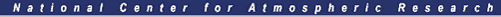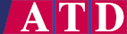#Calculating Windchill Values

#### [Windchill Tables][Windchill Introduction]

Two of the more common formulations for windchill were developed by Siple (1945) and Court (1948). Court modified Siple's formulation only slightly, using "certain observed values" that were apparently ignored by Siple. Both formulations have the same algebraic structure, but with different constants; both provide an equation for heat loss (H) from an exposed surface. Selection of a "baseline" condition of windspeed and temperature allows solving for a windchill temperature at different conditions.

```	H  =  ( A + B * sqrt(S) + C * S ) dT

where:
H  == Heat Loss, kg cals/m²/hr
dT == temperature difference (°C), between assumed
skin temperature (33°C) and ambient temperature
S  == windspeed, m/s

and the constants, A, B, and C are:

Siple	Court
A	10.45	 9.00
B	10.00	10.90
C	-1.00	-1.00
```
In a practical sense, with the following definitions, use the following formulas for calculating windchill (Siple's and Court's formulas, rearranging terms and substituting for unit conversions):

Twc == windchill temperature
T == ambient temperature
S == windspeed

For °C and m/s (baseline conditions of 33°C and 1.8 m/s):

```Siple:     Twc = 33  +  ( T - 33 ) ( .474  + .454 sqrt(S)  - .0454 S )

Court:     Twc = 33  +  ( T - 33 ) ( .550  + .417 sqrt(S)  - .0454 S )

for S >= 1.79 m/s
T < 33 °C
```

For °F and mph (baseline conditions of 91.4°F and 4 mph):

```Siple:     Twc = 91.4  +  ( T - 91.4 ) ( .474 + .304 sqrt(S) - .0203 S )

Court:     Twc = 91.4  +  ( T - 91.4 ) ( .550 + .279 sqrt(S) - .0203 S )

for  S >= 4 mph
T < 91.4 °F

Test (Siple): S=20mph, T=20 °F ---> Twc = -10.5 °F
```

And finally, for folks who prefer °F and knots (baseline conditions of 91.4°F and 3.47 knots):

```Siple:     Twc = 91.4  +  ( T - 91.4 ) ( .474 + .326 sqrt(S) - .0234 S )

Court:     Twc = 91.4  +  ( T - 91.4 ) ( .550 + .299 sqrt(S) - .0234 S )

for  S >= ~3.5 knots      (note: 1 mph = .869 knots)
T < 91.4 °F

```

### Some notes:

There is nothing "exact" about windchill: it is an approximation or estimate. Other indices of "physiological" or "perceptual" temperature exist.

There's some uncertainty concerning the "baseline" values to be used. However, the stated values are likely close enough, and since windchill is only a crude estimate, ...

### References:

Bair, Frank E., ed., 1992: The Weather Almanac, 6th edition. Gale Research, Inc., pp 140-143.

Court, A., 1948: Windchill. Bull. Amer. Meteor. Soc., 29, 487-493.

Siple, P.A., and C.F. Passel, 1945: Measurements of dry atmospheric cooling in subfreezing temperatures. Proc. Amer. Phil. Soc., 89, 177-199.

Steadman, R.G., 1971: Indices of windchill of clothed persons. J. Appl. Meteor., 10, 674-683.

Document Creation: Bob Rilling, / NCAR Atmospheric Technology Division
Created: 12-Feb-1996